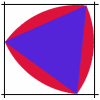#### You may also like### Marbles

I start with a red, a green and a blue marble. I can trade any of my marbles for two others, one of each colour. Can I end up with five more blue marbles than red after a number of such trades?### Rolling Triangle

The triangle ABC is equilateral. The arc AB has centre C, the arc BC has centre A and the arc CA has centre B. Explain how and why this shape can roll along between two parallel tracks.### Nine Colours

Can you use small coloured cubes to make a 3 by 3 by 3 cube so that each face of the bigger cube contains one of each colour?

# More Marbles

##### Age 11 to 14Challenge Level

This problem follows on from Marbles

Suppose I start with four marbles, one of each colour red, blue, green and yellow.When I trade marbles I give away one marble of any colour and I receive one marble of each of the other three colours.

Can I trade marbles so that I will have exactly two marbles of each colour?

Can I have equal even numbers of each colour after a number of trades?

What about ending up with 5 marbles of each colour?

Is this possible and if so how many trades does it take?

Is it possible to end up with the same odd number of each colour for any odd number?